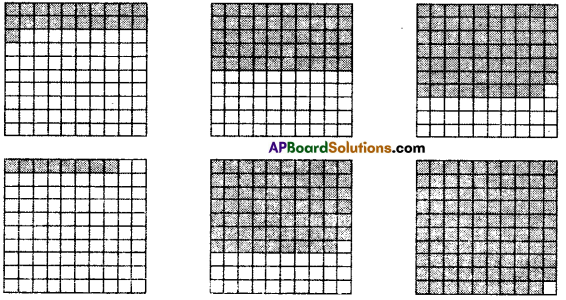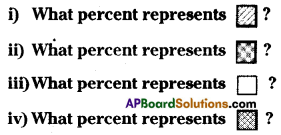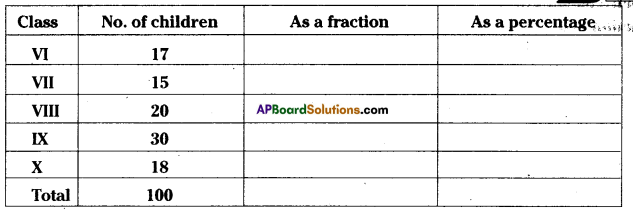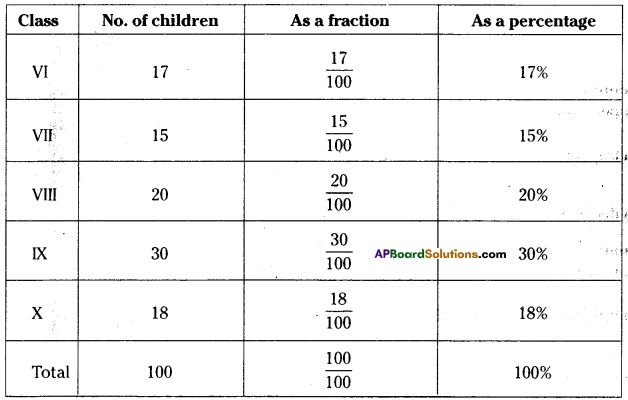# HSSlive: Plus One & Plus Two Notes & Solutions for Kerala State Board

## AP Board Class 7 Maths Chapter 6 Ratio Applications InText Questions Textbook Solutions PDF: Download Andhra Pradesh Board STD 7th Maths Chapter 6 Ratio Applications InText Questions Book AnswersAP Board Class 7 Maths Chapter 6 Ratio Applications InText Questions Textbook Solutions PDF: Download Andhra Pradesh Board STD 7th Maths Chapter 6 Ratio Applications InText Questions Book Answers

## Andhra Pradesh State Board Class 7th Maths Chapter 6 Ratio Applications InText Questions Books Solutions

 Board AP Board Materials Textbook Solutions/Guide Format DOC/PDF Class 7th Subject Maths Chapters Maths Chapter 6 Ratio Applications InText Questions Provider Hsslive

2. Click on the Andhra Pradesh Board Class 7th Maths Chapter 6 Ratio Applications InText Questions Answers.
3. Look for your Andhra Pradesh Board STD 7th Maths Chapter 6 Ratio Applications InText Questions Textbooks PDF.
4. Now download or read the Andhra Pradesh Board Class 7th Maths Chapter 6 Ratio Applications InText Questions Textbook Solutions for PDF Free.

## AP Board Class 7th Maths Chapter 6 Ratio Applications InText Questions Textbooks Solutions with Answer PDF Download

Find below the list of all AP Board Class 7th Maths Chapter 6 Ratio Applications InText Questions Textbook Solutions for PDF’s for you to download and prepare for the upcoming exams:

Try This

Question
Think of some real life situations in which you have to compare quantities in the form of a ratio. (Page No. 111)
Solution:

1. Boys and girls ratio in a class.
2. Number of matches won and lost by India at World Cup.
3. Number of students come by bycycle and on foot.

Do This

Question 1.
40 benches are required to seat 160 Sfciidents. How many benches will be required to seat 240 students at the same rate ? (Page No. 116)
Solution:
Number of benches required to seat 160 students= 40
On one bench, the number of students that can be seated = 16040 = 4 . 40
∴ For 240 students the number of benches required = 2404= 60

Question 2.
When a Robin bird flies, it flaps wings 23 times in 10 sec. How many times will it flap its wings in 2 minutes ? (Page No. 116)
Solution:
In 10 sec. Robin bird flaps for 23 times.
In 1 sec Robin bird flaps 2310 times
In 2 minutes = 2 x 60 = 120 sec Robin bird flaps for = 2310 x 120 = 276 times

Question 3.
The average human heart beats at 72 times per minute. How maiiy times does it beat in 15 seconds ? How many in 1 hour ? How many in a day ? (Page No. 116)
Solution:
Number of beats per 1 minute (60 seconds) = 72 times
Number of beats per 1 second = 7260=65 times
Number of beats per 15 seconds = 65 x 15 =18 times
∴ Number of beats per 1 hour = 65 x 60 x 60 = 4320 times
∴ Number of beats per 1 day = 4320 x 24 = 103680 times.

Try This

Question 1.
Population of our country as per 2011 census is about 12 x 108 (120,00,00,000). If the popu-lation of our country increases by 3% every year what will be the population by 2012 ? (Page No. 126)
Solution:
Population in 2011 = 12 x 108
It increases by 3%
Population in 2012 = (100 + 3) % of 2011
= 103% of 12 x 108
= 103×12×108100
= 1236×108100
= 12.36 x 108 .

Question 2.
i) Can you eat 75% of a dosa ? (Page No. 126)
Solution:
Yes.

ii) Can the price of an item go up by 90% ?
Solution:
Yes.

iii) Can the price of an item go up by 100% ?
Solution:
Yes.

Do This

Question 1.
Find the interestonasum of825O for3yearsat the rate of 8%perannum. (Page No. 138)
P = ₹ 8250 R = 8% T = 3 years
Interest =P x R% x T
I = 8250 x 8100 x 3 .
Interest = 198000100 = 1980

Question 2.
₹ 3000 is lent out at 9% rate of interest. Find the Interest which will be reèieved at the end of 2½ years. (Page No. 138)
Sol. P = ₹3000 R = 9% T = 2½ years = 52,
Interest = P x R% x T
3000 x 9100×52 = ₹675

Do This

Question
Given below are various grids of 100 squares. Each has a different number of squares coloured.
In each case, write the coloured and white part in the form of a 1) Percentage 2) FractIon and 3) Decimal (Page No. 121)Solution:
I) Coloured squares = 21
as a percentage 21%
as a fraction = 21100

ii) Coloured squares = 50
as a percentage = 50%
asafraction = 50100=12
as a decimal = 50100 = 0.50

iii) Coloured squares = 69
as a percentage = 69%
as a fraction = 69100
as a decimal = 69100 = 0.69

iv) Coloured squares = 8
as a percentage = 8%
as a fraction = 8100=225
as a decimal = 8100 = 0.08

v) Coloured squares = 70
as a percentage = 70%
as a fraction = 70100
as a decimal = 70100 = 0.70

vi) Coloured squares = 99
as a percentage = 99%
as a fraction = 99100
as a decimal =99100 = 0.99

Question 2.
Look at the grid paper given below. It Is shaded In various designs. Find the percentage of each design (Page No. 122)Solution:
(i) 19%
(ii) 40%
(iii) 34%
(iv) 7%

Question 3.
The strength particular of a school are given below. Express the strength of each class as a fraction, percentage of total strength of the school. (Page No.122)Solution:Try This

Question 1.
The C.P of 12 mangoes is equal to the selling price (S.P) of 15 mangoes. Find the loss percent. (Page No. 128)
Solution:
C.P. of 12 = S.P of 15
∴ Loss = 3
Loss percentage = 315 x 100% = 20%

## Andhra Pradesh Board Class 7th Maths Chapter 6 Ratio Applications InText Questions Textbooks for Exam Preparations

Andhra Pradesh Board Class 7th Maths Chapter 6 Ratio Applications InText Questions Textbook Solutions can be of great help in your Andhra Pradesh Board Class 7th Maths Chapter 6 Ratio Applications InText Questions exam preparation. The AP Board STD 7th Maths Chapter 6 Ratio Applications InText Questions Textbooks study material, used with the English medium textbooks, can help you complete the entire Class 7th Maths Chapter 6 Ratio Applications InText Questions Books State Board syllabus with maximum efficiency.

## FAQs Regarding Andhra Pradesh Board Class 7th Maths Chapter 6 Ratio Applications InText Questions Textbook Solutions

#### Can we get a Andhra Pradesh State Board Book PDF for all Classes?

Yes you can get Andhra Pradesh Board Text Book PDF for all classes using the links provided in the above article.

## Important Terms

Andhra Pradesh Board Class 7th Maths Chapter 6 Ratio Applications InText Questions, AP Board Class 7th Maths Chapter 6 Ratio Applications InText Questions Textbooks, Andhra Pradesh State Board Class 7th Maths Chapter 6 Ratio Applications InText Questions, Andhra Pradesh State Board Class 7th Maths Chapter 6 Ratio Applications InText Questions Textbook solutions, AP Board Class 7th Maths Chapter 6 Ratio Applications InText Questions Textbooks Solutions, Andhra Pradesh Board STD 7th Maths Chapter 6 Ratio Applications InText Questions, AP Board STD 7th Maths Chapter 6 Ratio Applications InText Questions Textbooks, Andhra Pradesh State Board STD 7th Maths Chapter 6 Ratio Applications InText Questions, Andhra Pradesh State Board STD 7th Maths Chapter 6 Ratio Applications InText Questions Textbook solutions, AP Board STD 7th Maths Chapter 6 Ratio Applications InText Questions Textbooks Solutions,
Share: